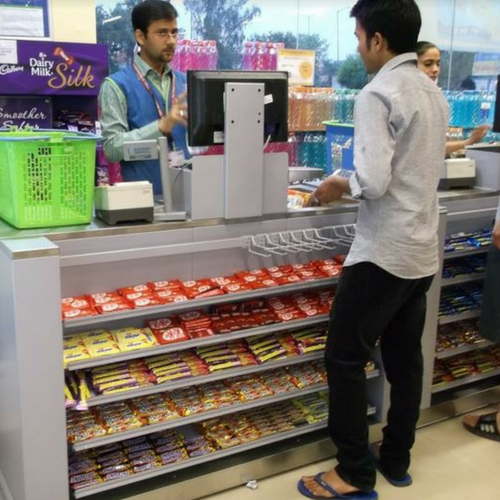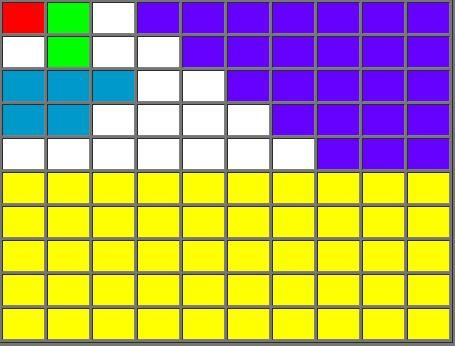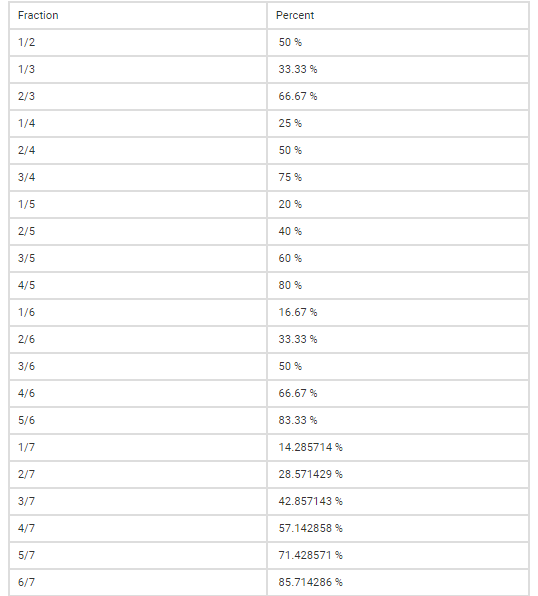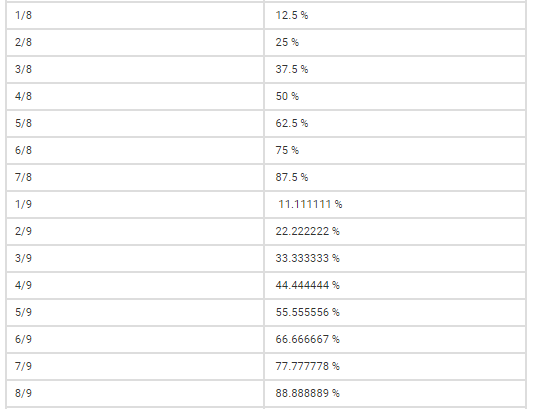Top

# Percentage

Aptitude(Numeracy & Reasoning)- 21st century literacies

Lesson Plan

Objective:

• To help students learn to express a number, fraction or ratio out of every hundred. Students get to learn how percentage facilitates the expression of huge data in its simplest form. Participants would be able to learn the basics of percentage and their usage in daily life.

• To enable learners to solve questions of any kind related to percentage. Students will be able to deduce solutions and develop an ability to understand the concept of percentages and its practical applications in daily life.

Total Time: 90 Minutes

Instructor Note: This unit would deal with the basic nuances of the ever important topic Percentage. Owing to its importance, it becomes inevitable to form a strong foundation and holistic learning of the same. Learners get to understand basic concepts of percentage and its practical usage. It would enable them to express a number, fraction or ratio out of every hundred. Participants get to learn how percentage facilitates the expression of huge data in its simplest form. Trainer is required to deliver this unit under an engaging environment by using practical examples and competitive activities.

(After explanation of concept & practice) Display/distribute handout consisting of questions. The Foundation section will compromise 4 questions. Instruct students that they will be given 8 minutes to discuss among themselves & solve these 4 questions, whichever group solves maximum problems wins maximum points on the points table (refer opening protocol). Award 5 marks for each correct answer given by a group. Move around the class to check procedures used by students to solve problems. Once time is up, provide solutions and explanations for each question.

Switch to moderate level. This section comprises 3 questions and cumulative time allotted will be 10 minutes. All other instructions remain the same.

Switch to HOTS. This section comprises 3 questions and cumulative time allotted will be 15 minutes. All other instructions remain the same.

Opening Protocol:

• Greet the participants.

• Take attendance.

• Form groups of participants with each group comprising of 4 members.(For entire session)

• Draw points table on board and ask participants to name their group with some fancy names like “Aptitude thunders”, “Knights of Mathematics”, “Fantastic Statisticians” etc.

• Inform the participants that the group which tops the points table will be rewarded at the end of session.

• Recap the previous session by asking 1-2 participants to share with the class what they learnt and did in the previous session.

3 Minutes

Opening Activity:

• Alright everyone! Before we begin, Let me ask you, how many of you like it if you are offered some off/discount on your purchase? (Raise a few students and ask what products they would purchase, if offered a discount).

• Okay! It seems all of you are excited to buy maximum products during a sale. Before that, Let me see how many of you can grab the best deals during a sale. Look at the case below:• Your local supermarket has a special price reduction this week.  When you go to the cash counter you are offered a choice of ”10% off or Rs.10 off on your total purchase”.

• Will your decision be the same in the following situations?

(I) Your shopping amounts to Rs.70/-

(II) Your shopping amounts to Rs.120/-

(III) your shopping amounts to Rs.200/-

(IV) Your shopping amounts to Rs.11/-

• Each group has to discuss and calculate which of the above captioned offers would be appropriate in given situations in order to make a purchase in your favor. You will be awarded points with respect to the right answers. Groups will also be rewarded points on their speed of calculation (rank in giving answer).

• Trainer’s role- Award points and explain the calculation.

• Now, Do you all think you would have made a purchase in your favor if you were unaware of the percentage? No! This topic plays a vital role in taking the right decisions during a transaction in our daily life. Let us move forward to understand the concept of percentage and practice few advance problems to enable ourselves to grasp this topic as a skill set.

10 Minutes

Section 1: Understanding Percentages.

• Percent comes from the Latin word “per centum”. The Latin word “centum” means 100, so “per centum” means “for each hundred”. Percent is denoted with a symbol “%”. For instance, 1 percent can be written as 1%, and it is the same as the fraction 1/100, Ten percent can be written as 10%, which is the same as the fraction 10/100.

• When we say percent, we mean a part of the whole where whole is considered as hundred. Look at the grid below with 100 cells to understand better.

• Each cell is equal to 1% of the whole (the red cell is 1%).

• Two cells are equal to 2% (the green cells).

• Five cells are equal to 5% (the blue cells).

• Twenty-five cells (purple cells) are equal to 25% of the whole or one quarter (¼).

• Fifty cells (yellow cells) are equal to 50% of the whole or half (½).• Now, Can you calculate how many unshaded (white) cells there are? What is the percentage of unshaded cells? There are three ways to work this out. Look at the following ways:

• Count the white cells. There are 17 of them. Out of 100 cells, 17% are therefore white.

• Add up the number of other cells and deduct them from 100. There is one red cell, two green, five blue, twenty five purple, and fifty yellow. That adds up to 83. So, (100−83) = 17. Again, out of 100 cells, 17 are white, or 17%.

• Divide the value of white cells by total value and then multiply the resultant to 100. Percentage= (Value of white cells/Total value)×100 OR 17/100 × 100 = 0.17 × 100 = 17 or 17%.

• Percentage is textually written as, Percentage= (Part/Whole) × 100. Now, this part & whole can be any value, but when we convert this ratio in percentage, we mean to define the resultant out of every hundred. This gives us freedom to express complex calculations in simple form. For example, If I say 20% of my shirt was torn, a person listening to this will get an idea about portion of shirt that was torn without even knowing actual length of the shirt and length of the portion that was torn because when I said 20% of my shirt was torn, he considered the whole shirt as hundred cells and imagined that 20 cells out of it were torn. Therefore, we can easily communicate in terms of percentage without expressing detailed calculations.

Section 2: Conversion of fraction into percentages and vice versa.

• To convert a fraction into percentage, just multiply the given ratio by 100, the result obtained will be in terms of percentage. Example: To express 2/3 in terms of percentage, we multiply the given ratio by 100 or (2/3) × 100 = 66.66%.

• Similarly, if we want to convert a number given in terms of percentage, we divide the number by 100. Example: To express 40% in terms of ratio, we divide 40 by 100 or (40/100) = 2/5.

• Trainer’s role- Explain to the students why we multiply and divide by 100 while converting from ratio to percentage & percentage to ratio respectively through unitary method. Give a few fractions to convert into percentage & vice versa to check understanding of concept.

• Now, look at the percentage-fraction conversion chart below. This will help you solve problems quickly.Section 3: Percentage increase or decrease.

• Percentage increase is the ratio of value increased to the original value and multiplied by 100. It is expressed in percentage. If there is an increase in the value of anything, then there is an increase in percentage.

• Value Increased   × 100 = Percentage Increase.   Original Value

• Let us understand this with an example. If your salary is Rs.50,000/- per month and one day your company’s HR walks in and informs you that you are promoted to senior management and your new salary will be Rs.60,000/-. What is the percentage increase or hike on your salary?

• Solution:  We can clearly see that there is an increase in your salary which is Rs.10,000/- . So, what will be the percentage increase? Percentage increase as defined above can be written as (10000/50000) * 100 which is equal to 20%. So you received a 20% hike on your current salary.

• Percentage decrease is the ratio of value decreased to the original value and multiplied by 100. It is expressed in percentage. If there is a decrease in the value of anything, then there is a decrease in percentage.

• Value Decreased × 100 = Percentage Decrease.   Original Value

• Let us understand this with an example. Last month your total expenditure was Rs.20,000/-. However, this month you have managed your expenses well and your total expenditure was Rs.15,000/- . What is the percentage decrease on your expenditure?

• Solution: We can clearly see that there is a decrease in your expenditure as compared to the previous month which is Rs.5000/-. So, what percentage will decrease? Percentage decrease as defined above can be written as (5000/20000) * 100 which is equal to 25%. So you reduce your expenses by 25%.

• An important point to note while calculating percentage increase or decrease is that the value increased or decreased is always calculated on original value and not on resultant value. This is a common mistake people make.

Section 4: Understanding the concept of multiplying factor for quick calculations.

• Let’s learn a unique approach to ease the calculation while solving percentage increase or decrease. This will also help you in solving general problems related to percentages very quickly.

• What do we mean when we say that a value has increased by 20%? As discussed earlier, we consider the total value of anything or it’s whole part as hundred or 100%. Now, if we say that this whole value has increased by 20%, we mean that a value which was 100% earlier has now increased by 20% or it has become 120% of the original value. 120% can be further written as 120/100 or 1.2. This “1.2” works out to be your multiplying factor. Multiplying 1.2 to your original value will give you the increased value.

• What do we mean when we say that a value has decreased by 20%? We mean that a value which was 100% earlier has now decreased by 20% or it has become 80% of the original value. 80% can be further written as 80/100 or 0.8. This “0.8” is your multiplying factor. Multiplying 0.8 to your original value will give you the decreased value.

• Using the concept of multiplying factors will help you save time from lengthy calculations through formulas. Let us understand this through a few examples.

•  If the current salary of an employee working in ABC company is Rs.24000/-. What will be his new salary if he received a hike of 20% on his salary?

Solution: According to the given question, the employee gets a hike of 20% on his salary. So, according to formula :

Value Increased  × 100 = Percentage Increase

Original Value

Value increased = (20 × 24000)/ 100 or Rs.4800/- or you could simply calculate 20% of 24000= 4800 (without using formula).

New salary of the employee will be 24000+4800 = Rs.28,800/-

• Can we make this simpler? Yes! By using the concept of multiplying factors. Here you go.

 24000 × 1.2 = 28,800.

Did we just get the answer in a single line? How did we do that? Simple! Salary of the employee was Rs.24000/- which can be considered as 100% of his salary as this amount represents the whole part of his salary. Now according to question, his salary increased by 20% which means now his salary is 120% of the original salary or we can express it as:

(120% of 24000) or 24000 × (120/100) or 24000 × 1.2.

• Let’s quickly solve a few problems. Groups will be awarded points on right answers and their ranking in solving problems. You will be given 10 minutes to solve the following problems.

• Increase 5000 by 23%.

• Increase 200 by 200%.

• Increase 17400 by 12%.

• Decrease 2800 by 13%

• Decrease 5000 by 20%.

• Decrease 12000 by 15%.

• Express ¾ in terms of percentage.

• Express 7/8 in terms of percentage.

• Express 15/14 in terms of percentage (round off the value)

• Express 125% in terms of fraction.

• Express 160% in terms of fraction.

• Express 285% in terms of fraction.

• Trainer’s role- Instruct students to discuss among their group members and help each other to solve the above problems. Award points to each group and subsequently solve the questions by using concepts discussed above.

Section 5: Few tricks to calculate faster

• Splitting the percentage into parts.

• Example: Find 51% of 128.

Solution: 51% of 128= (50+1)% of 128= 50% of 128 + 1% of 128 = 64 + 1.28 = 65.28

• Interchanging the percentage value and the number.

• Example: Find 39% of 12.5.

Solution: 39% of 12.5 = 12.5% of 39 = 1/8 × 39 = 4.875

30 Minutes

 Section 6: Foundation. The population of a town is 352800. If it increases at the rate of 5% per annum, then what will be its population 2 years hence. Also, find the population 2 years ago. An agent gets a commission of 2.5% on the sales of cloth. If on a certain day, he gets Rs.12.50 as commission, the cloth sold through him on that day is worth? If 35% of a number is 175, then what percent of 175 is that number? If the numerator of a fraction is increased by 120% and denominator is also increased by 350% then the fraction becomes 11/27, What was the fraction? Section 7: Moderate. If the price of petrol is increased by 20%, by what percentage should the consumption be decreased by the consumer, if the expenditure on petrol remains unchanged? In a certain school, 20% of students are below 8 years of age. The number of students above 8 years of age is 2/3 of the number of students of 8 years age which is 48. What is the total number of students in the school? In an examination 20% failed in Hindi, 25% failed in English and 7% failed in both subjects. What will be the percentage of students who failed in at least one of the subjects? Section 8: HOTS- High order thinking skills. Sweta invested Rs.10000/- in a scheme exactly three years ago. The value of the investment increased by 10% during the first year, increased by 5% during the second year, and decreased by 10% during the third year. What is the value of her investment today? ABC private limited appoints a sales representative on the basic salary of Rs.1200/- per month and with the condition that for every sales of Rs.10000 above Rs.10000, he will get 50% of basic salary and 10% of the sales as reward. There is no incentive for the first Rs.10000 of sales. What should be the value of sales if the sales representative wants to earn Rs.7600/- in a particular month? In Delhi, 60% of the registered voters are BJP-supporters and the rest are congress supporters. In a mayoral race, if 75% of the registered voters who are BJP-supporters and 20% of the registered voters who are congress supporters are expected to vote for candidate X, what percent of the registered voters are expected to vote for candidate X? 8 Minutes Answers 1.388962,    320000 2. 500 3. 285.71 4. 5/6 10 Minutes Answers 1.(50/3)% 2. 100 3. 38% 15 Minutes Answers 1. 10,395 2. 50,000 3. 53% Exp Time 10 minutes Closing Activity: Thank you everyone! Now, all of you are clear with your concepts of percentage. You will be getting materials for practice that you have to solve by yourself at home and bring doubts, if any. You will be provided handouts with concepts discussed which will help you brush up and revive your concepts any time in future. Trainer needs to calculate total points accumulated on the points table and reward the winning group. In case of a tie between two or more groups, reward each of the winning groups. Okay, students! We will be ending this session with a famous quote. You miss 100 percent of the shots you don’t take. 1 Minute Closing Protocol:  Thank the participants for their time. Announce the next time you will be visiting them. Share the topic of the next session. Share the names of reward winning students with nodal officers and institution heads after the session. Post-session